### Home > CALC > Chapter 5 > Lesson 5.2.1 > Problem5-52

5-52.
1. Differentiate if possible. If the function is one we have not yet studied, say so and explain how you know. Be sure to use the appropriate notation. Homework Help ✎

1. y = x100 + 100x

2.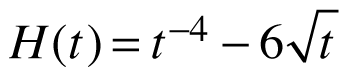3. y = x · 2x

4. y = x8 cos x

5. f(x) = x5 (x3 + π)

6.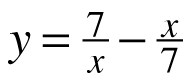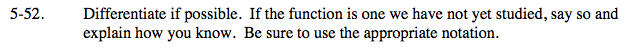You have the skills to find the derivative of five out of six of the problems below.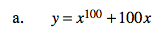Power Rule.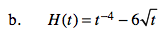Before differentiating, rewrite the square root with a fractional exponent.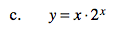Do you know how to find a derivative of 2x?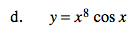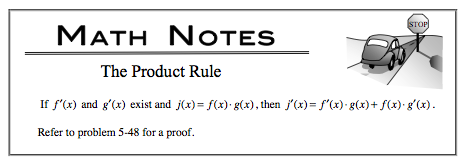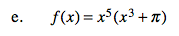Distribute first. Or use the Product Rule.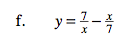Before differentiating, rewrite the first fraction with exponents.